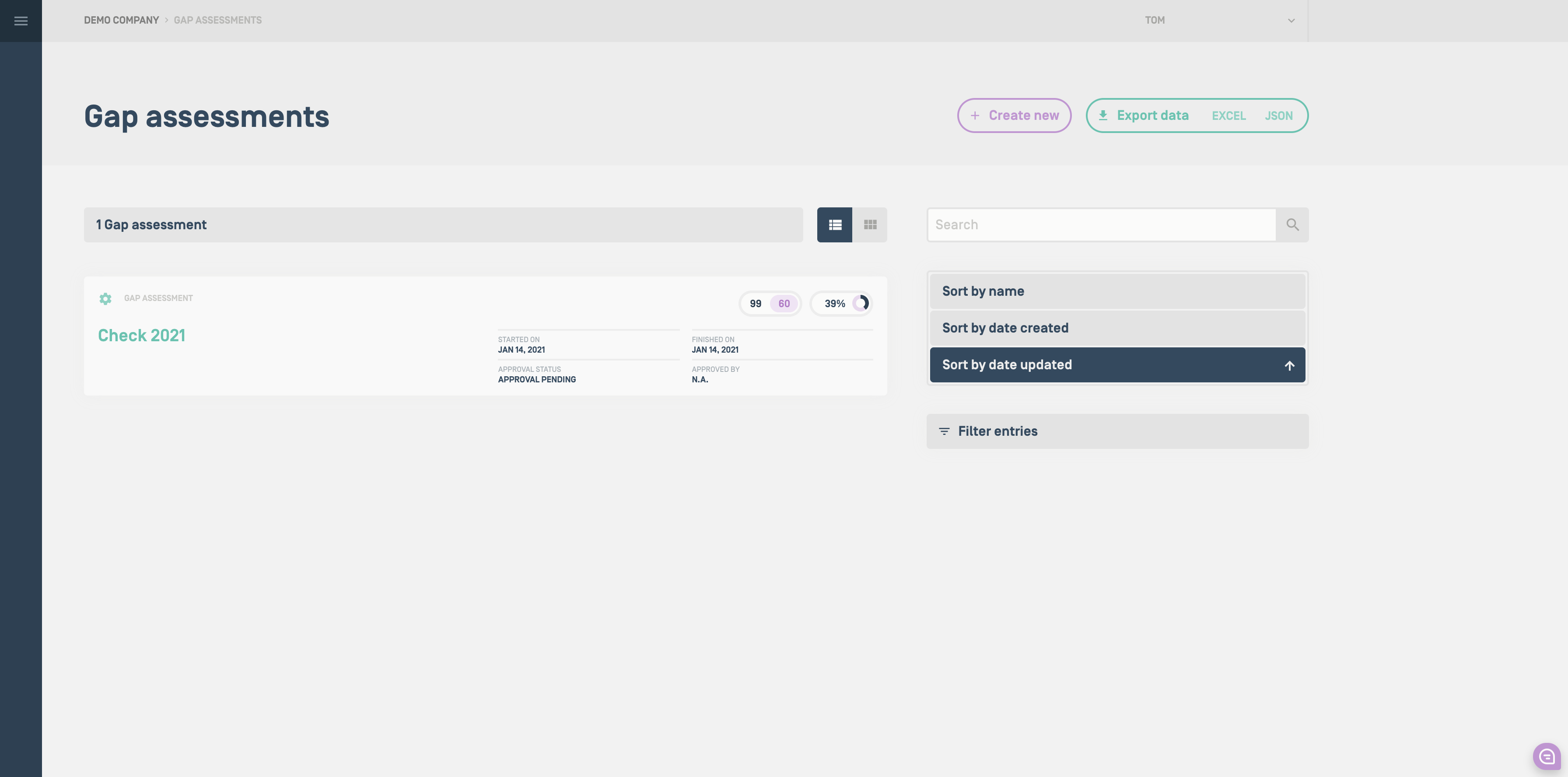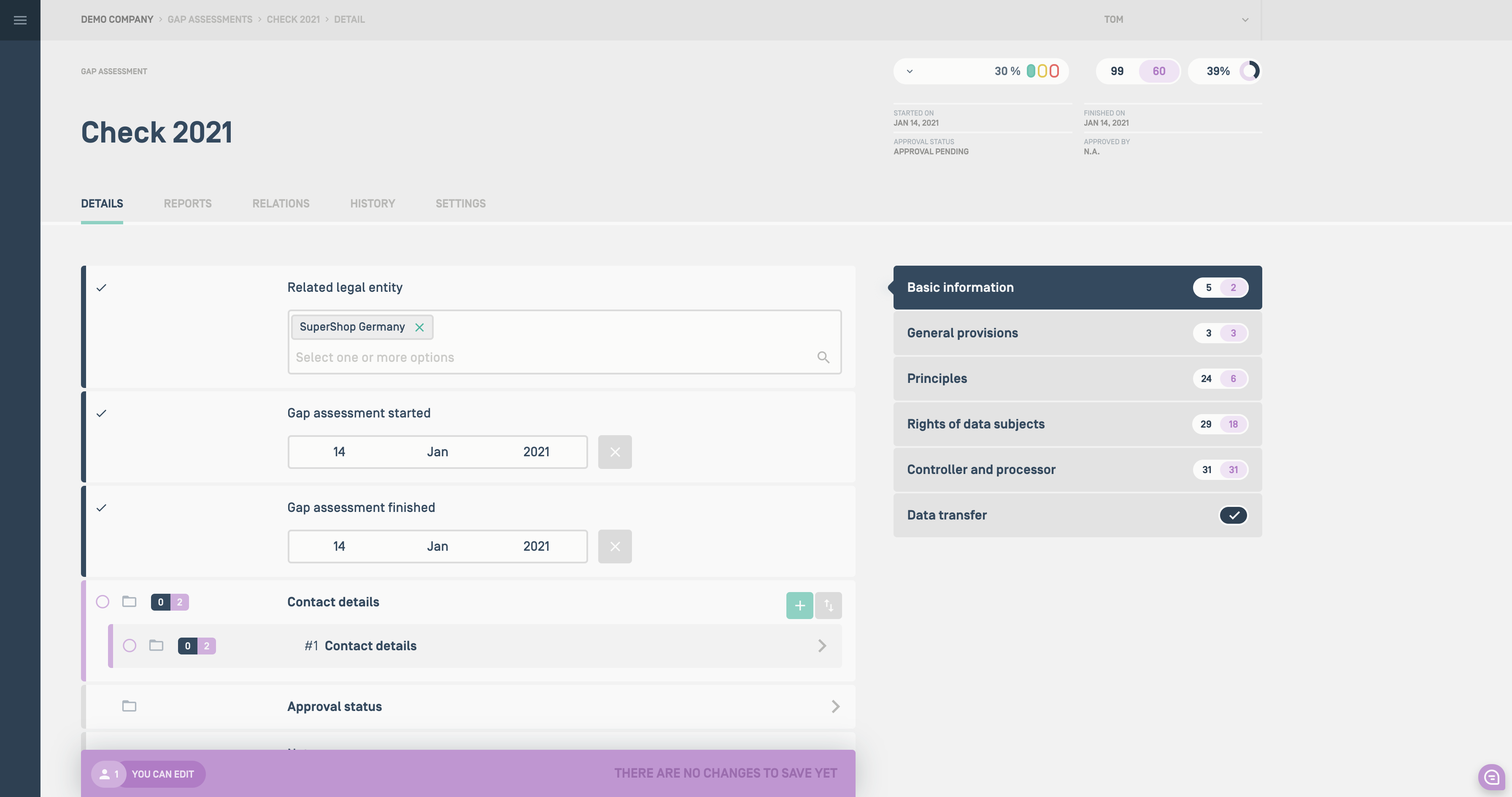Each object in ZOA has some summary statistics to inform you about the level of completeness and if there are data elements missing.

### Statistics on the overview pages

Each object on the overview pages shows three statistics:

• Number of required data fields / questions. In this example, there are 99 required questions to answer.
• Number of missing required data fields / questions. We still have to answer 60 questions in the example below.
• Same information as above expressed as a percentage of completeness number. As we have already answered 39 out of 99 questions, the percentage of completion is 39%.### Statistics on object pages

The same statistics as described above are also shown on the object pages in the upper right corner.